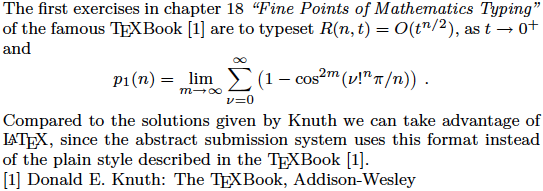# Contribution Submission

## Hints on the LaTeX notation

Math formulas and similar things can be created by the optional (i.e. it need to be activated for use) LaTeX notation in the submission system. Some very basic constructs are:

Typeset textLaTeX code
s-1s$^{-1}$
H2OH$_{2}$O

In a more complex example this could look like:

The first exercises in chapter 18 \textit{Fine Points of
Mathematics Typing''} of the famous \TeX{}Book  are to typeset
$R(n,t)=O(t^{n/2})$, as $t\to0^+$ and
\begin{displaymath}
p_1(n)=\lim_{m\to\infty}\sum_{\nu=0}^\infty
\left(1-\cos^{2m}(\nu!^n\pi/n)\right)\,.
\end{displaymath}
Compared to the solutions given by Knuth we can take advantage
of \LaTeX, since the abstract submission system uses this format
instead of the plain style described in the \TeX{}Book .
\scCite{1}{Donald E. Knuth: The \TeX{}Book, Addison-Wesley}


The submission system will create the following output out of this text:The system allows to use various standard LaTeX constructs, which are covered in detail by the relevant literature. A good online reference is the page of the LaTeX project. Of special interest might be the section documentation where among other things a link to The (not so) Short Introduction to LaTeX2e can be found. This document provides a short overview about typesetting of mathematical symbols, formulas and others features of the LaTeX system.

Note that activating the LaTeX notation in the form leads to a special meaning of certain characters which thus need to be replaced.

DPG-Physik > DPG Conferences > SKM23 > Forms